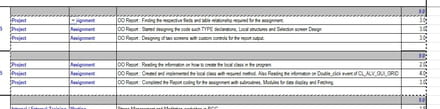# To copy data to other workshet by suming the value based on co

Closed
-
Posts
2
Registration date
Wednesday July 15, 2015
Status
Member
Last seen
July 17, 2015
-
Hello,

I am having table like below with large data in DATA.xlsx filePls see attached file for table details

My requirement is to select the data available in the sheet and sum the hours values base on on col-A(i.e. Project values column) if col-a is equal to "Project" and paste this sum to other excel file.

I had tried to use the above code but copies all the value instead of specific.
Please some help me out for this solution.

Posts
2
Registration date
Wednesday July 15, 2015
Status
Member
Last seen
July 17, 2015

Please use the inbuilt functionality of SUMIF to get the required result value and then by using for and if loop assign the values. e.g
sample code is as below and repeat the same for more others.:

Sub weeklydata()
Dim i As Integer, stng As String, lastrow As Integer
Dim var1 As Single, var2 As Single, var3 As Single, var4 As Single
Dim worksh As Integer, x As Integer

Workbooks("Report.xlsm").Sheets("Final Weekly Report").Activate

lastrow = ActiveSheet.Range("A" & Rows.Count).End(xlUp).Row

For i = 99 To lastrow
'If Cells(i, 1) = "WEEK 14" Then

If Cells(i, 2) = "Kumar" Then

Worksheets("CW14").Select
Sheets("CW14").Activate
Range("c12:f38").Select

var1 = WorksheetFunction.SumIf(Range("C13:c37"), "Project", Range("f13:f37"))
var2 = WorksheetFunction.SumIf(Range("C13:c37"), "Enabling", Range("f13:f37"))
var3 = WorksheetFunction.SumIf(Range("C13:c37"), "Internal / External Training", Range("f13:f37"))
var4 = WorksheetFunction.SumIf(Range("C13:c37"), "Leave/Out of Office", Range("f13:f37"))

'ActiveWorkbook.Save
ActiveWorkbook.Close

Cells(i, 3).Value = var1
Cells(i, 4).Value = var2
Cells(i, 5).Value = var3
Cells(i, 6).Value = var4
ActiveWorkbook.Save

var1 = 0
var2 = 0
var3 = 0
var4 = 0
Exit For
End If
Next x
endsub